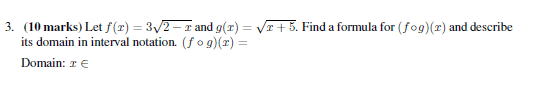# Composite function help! ? - Thanks!

nukeman

## Homework Statement

Here is the problem:## The Attempt at a Solution

I need help RIGHT from step one.

Now, step one I would suppose I need to evaluate g(x) ? Which, would be x must be greater than or equal to -5. Correct?

Then what?

Nessdude14
The first step is to write the function f(g(x)). What this means is that for the function f(x), you substitute x=g(x). Then, you need to find the domain of this f(g(x)) function.

nukeman
Ok, so first thing I would do is write in the g(x) into the f(x) ?

So, it turns into: f(x) is 3(2-(x + 5)^1/2)^(1/2)

? That cant be correct :(

Nessdude14
Yep, that's correct, except it's f(g(x)), not f(x).

nukeman
Really? lol cool.

Ok, so then after that, what do I do? This is where I get very confused.

Nessdude14
Neither square-root can have a negative number inside, so to find the domain, you need to find the intervals of x that make both the inner and outer square-roots positive.

In other words, x needs to satisfy the conditions:
$\displaystyle x+5≥0$
and
$\displaystyle 2-\sqrt{x+5}≥0$

nukeman
So this is all I do to find the formula, as asking in the question. f(x) is 3(2-(x + 5)^1/2)^(1/2)

x + 5 >= 0 would just be [0, infinity) right? is that what you mean?

Now sure how you got the 2nd one? what about the 3?

Nessdude14
So this is all I do to find the formula, as asking in the question. f(x) is 3(2-(x + 5)^1/2)^(1/2)

x + 5 >= 0 would just be [0, infinity) right? is that what you mean?

Now sure how you got the 2nd one? what about the 3?

The 3 is on the outside of the 2nd square root. You're just trying to make the inside of the square root larger than 0, so the 3 can be disregarded when finding the domain.

In order to solve the inequalities, you treat it just like any other equation. To solve the first one, you subtract 5 from both sides to get:

$\displaystyle x+5 ≥ 0$

$\displaystyle x ≥ -5$

Hopefully that gives you an idea how to solve the second inequality. After you find the solution to both of those, your domain will be the x values that satisfy both inequalities.

f(x) is 3(2-(x + 5)^1/2)^(1/2)
Also, remember that the composite function is not f(x), but f(g(x)). (also written as $(f \circ g)(x)$)

Last edited:
nukeman
I think I got it....

[-5, -1]

?

Saitama
I think I got it....

[-5, -1]

?

Yes, that's right.

Nessdude14
Yep, I think that's right.